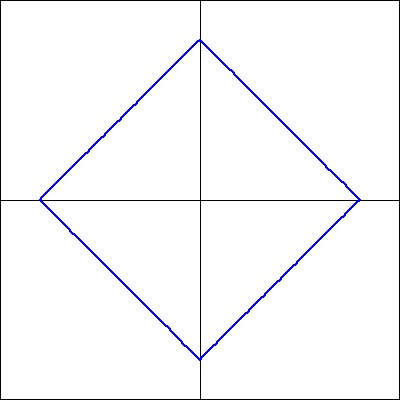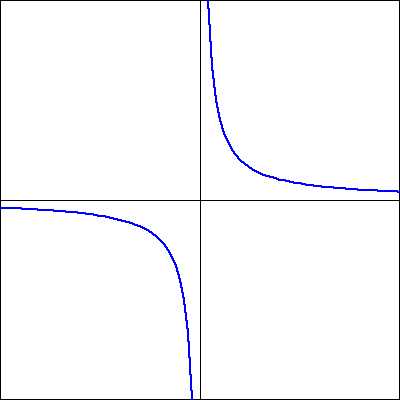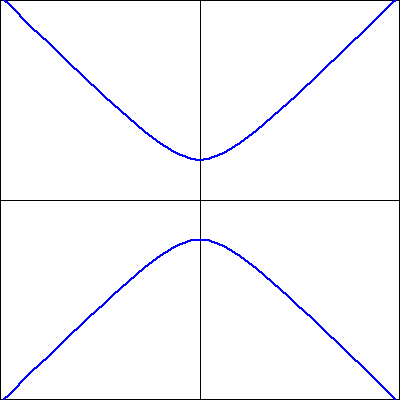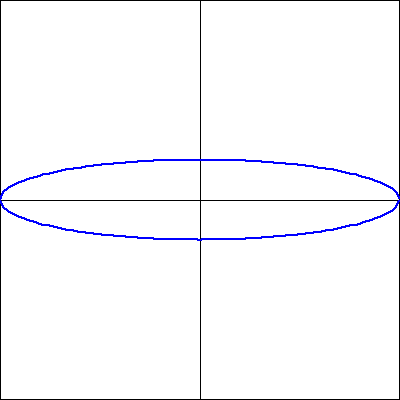This is similar to Problem 3 from your Section 4.1 worksheet.

Determine whether the following graphs represent $y$ as a function of $x.$ In each graph, the $x$-axis is horizontal and the $y$-axis is vertical.
1. Does the graph represent a function of $x$?2. Does the graph represent a function of $x$?3. Does the graph represent a function of $x$?4. Does the graph represent a function of $x$?Note: You can click on the graphs to enlarge the images.
Note: You only have three attempts at this problem.